# GMAT Math : DSQ: Calculating the equation of a line

## Example Questions

### Example Question #1 : Dsq: Calculating The Equation Of A Line

Find the equation of linear function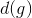given the following statements.

I)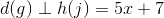II)intercepts the x-axis at 9.

Statement II is sufficient to answer the question, but Statement I is not sufficient to answer the question.

Either statement is sufficient to answer the question.

Both statements are needed to answer the question.

Statement I is sufficient to answer the question, but Statement II is not sufficient to answer the question.

Both statements are needed to answer the question.

Explanation:

To find the equation of a linear function, we need some combination of slope and a point.

Statement I gives us a clue to find the slope of the desired function. It must be the opposite reciprocal of the slope of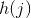. This makes the slope ofequal to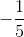Statement II gives us a point on our desired function,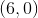.

Using slope-intercept form, we get the following: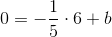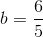So our equation is as follows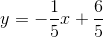### Example Question #2 : Dsq: Calculating The Equation Of A Line

There are two lines in the xy-coordinate plane, a and b, both with positive slopes. Is the slope of a greater than the slope of b?

1)The square of the x-intercept of a is greater than the square of the x-intercept of b.

2) Lines a and b have an intersection at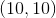Statement 1 alone is sufficient.

Statement 2 alone is sufficient.

Neither of the statements, together or separate, is sufficient.

Together the two statements are sufficient.

Either of the statements is sufficient.

Neither of the statements, together or separate, is sufficient.

Explanation: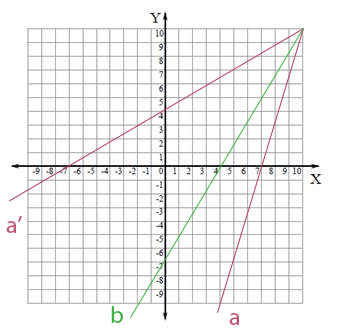Given that the square of a negative is still positive, it is possible for a to have an x-intercept that is negative, while still having a positive slope. The example above shows how the square of the x-intercept for line a could be greater, while having still giving line a a slope that is less than that of b.

### Example Question #3 : Dsq: Calculating The Equation Of A Line

Line j passes through the point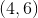. What is the equation of line j?

1) Line j is perpindicular to the line defined by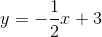2) Line j has an x-intercept ofNeither of the statements, separate or together, is sufficient.

Statement 2 alone is sufficient.

Either of the statements is sufficient.

Statement 1 alone is sufficient.

Together, the two statements are sufficient.

Either of the statements is sufficient.

Explanation:

Either statement is sufficient.

Line j, as a line, has an equation of the formStatement 1 gives the equation of a perpindicular line, so the slopes of the two lines are negative reciprocals of each other: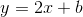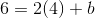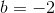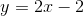Statement 2 allows the slope to be found using rise over run: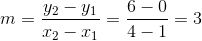Then, since the x-intercept is known: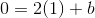### Example Question #4 : Dsq: Calculating The Equation Of A Line

Find the equation for linear function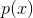.

I)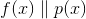and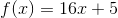II)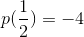Statement I is sufficient to answer the question, but statement II is not sufficient to answer the question.

Both statements are needed to answer the question.

Either statement is sufficient to answer the question.

Statement II is sufficient to answer the question, but statement I is not sufficient to answer the question.

Both statements are needed to answer the question.

Explanation:

Find the equation for linear function p(x)

I)andII)To begin:

I) Tells us that p(x) must have a slope of 16

II) Tells us a point on p(x). Plug it in and solve for b: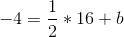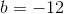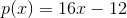### Example Question #5 : Dsq: Calculating The Equation Of A Line

Give the equation of a line.

Statement 1: The line interects the graph of the equation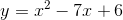on the-axis.

Statement 2: The line interects the graph of the equationon the-axis.

BOTH STATEMENTS TOGETHER provide sufficient information to answer the question, but NEITHER STATEMENT ALONE provides sufficient information to answer the question.

STATEMENT 2 ALONE provides sufficient information to answer the question, but STATEMENT 1 ALONE does NOT provide sufficient information to answer the question.

STATEMENT 1 ALONE provides sufficient information to answer the question, but STATEMENT 2 ALONE does NOT provide sufficient information to answer the question.

BOTH STATEMENTS TOGETHER do NOT provide sufficient information to answer the question.

EITHER STATEMENT ALONE provides sufficient information to answer the question.

BOTH STATEMENTS TOGETHER do NOT provide sufficient information to answer the question.

Explanation:

Assume both statements to be true. Then the line shares its- and-intercepts with the graph of, which is a parabola. The common-intercept can be found by settingand solving for: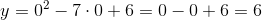,

making the-intercept of the parabola, and that of the line,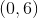.

The common-intercept can be found by setting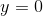and solving for: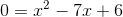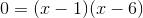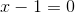, in which case, or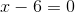, in which case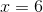,

The parabola therefore has two-intercepts,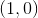and, so it is not clear which one is the-intercept of the line. Therefore, the equation of the line is also unclear.

Tired of practice problems?

Try live online GMAT prep today.